# 2nd PUC Statistics Question Bank Chapter 3 Time Series

Students can Download Statistics Chapter 3 Time Series Questions and Answers, Notes Pdf, 2nd PUC Statistics Question Bank with Answers helps you to revise the complete Karnataka State Board Syllabus and to clear all their doubts, score well in final exams.

## Karnataka 2nd PUC Statistics Question Bank Chapter 3 Time Series

### 2nd PUC Statistics Time Series Exercise Problems

Question 1.
Define cyclical variation.
Arrangement of statistical data in chronological order, in accordance with time is known as Time Series.

Question 2.
What is a Histogram?
A graphical representation of Time series data is called histogram

Question 3.
Define secular trend give an example.
The general tendency of the time series data to increase or to decrease or to remain constant during a long period of time is named as secular trend
Example:
Increase in the population of a country from past 7 years.
Decrease in the sales of a commodity for the past 5 years.Question 4.
Define seasonal variation. Give an example.
The regular, periodic and short term variation (less than a year) in a time series is defined as seasonal variation.
Ex: Increase in the sale of cotton clothes in summer.

Question 5.
Which index is used for the measurement of seasonal variation?
Seasonal indices

Question 6.
Define cyclical variation.
The oscillatory (up and down) movements in a series with period of oscillation more than one year are termed as Cyclical fluctuation. One complete period is called a Cycle.

Question 7.
What are the different phases in a business cycle?
Prosperity, Decline, Depression, Improvement.

Question 8.
Diagrammatically represent “Business cycle” with stages?Question 9.
Define random variation? Give an example.
Irregular or unpredictable variation which are beyond the control of human hand.
Example: Sudden increase in price of fuel due to war in Iran

Question 10.
Mention a cause of the following variations:
(i) Seasonal variation
(ii) Cyclical variation
(iii) Irregular variation
(i) Seasonal variation:
(a) Weather and climatic conditions
(b) Customs, traditions and preferences of people

(ii) Cyclical variation: Prosperity, recession, depression and recovery

(iii) Irregular variation: War, floods, strikes, lock outs, earthquakes

Question 11.
Which variation is predictable?
Regular variations like seasonal, cyclical, etc.Question 12.
Which variation is unpredictable?
Irregular variations.

Question 13.
Which components of Time-Series are associated with the following sentence?
(a) Fall in death rate due to advance in science
(b) An increase in employment during harvest season
(a) Fall in death rate due to advance in science-secular trend
(b) An increase in employment during harvest season – seasonal trend.

Question 14.
Name two methods of measuring trend?

1. Graphical method
2. Semi averages method
3. Method of Moving Averages
4. Method of Least Squares

Question 15.
State two conditions of least squares method of measuring trend.
1. The sum of the deviations of actual (y) and the trend values (ŷ) is zero, i.e., Σ(y – ŷ) = 0

2. The sum of the squares of the devation of actual (y) and the trend values (ŷ) is least,
i.e., Σ(y – ŷ)2 is least.

Question 16.
Write down the normal equations for fitting linear trend?
The straight line equation is
y = a + bx
The normal equations are
Σy = na + bΣx
Σxy = a Σx + b Σx2.

Question 17.
Explain the semi averages method of measuring trend.
In this method, the time series data is divided into two equal parts and averages are calculated for both the parts, are called semi averages. These averages are plotted on a graph, joined by a straight line and the end points are extended on either side, is the trend line, which indicates over all trend of the time series.

Question 18.
Explain the moving averages method of measuring trend.
Moving averages are the averages obtained by finding the arithmetic means of successive values of time series by taking 3/4/5 years at a time, by leaving the first and adding the next value.

These averages eliminates short term variations / fluctuations and gives the overall trend of the time series are called trend values (ŷ)

Question 19.
Explain with merits and demerits of the method of moving averages for measuring trend.
Merits:
(a) It is simple and easy to understand
(b) It is more flexible than other methods
(c) It is not only used for the measurement of trend but also for the measurement of seasonal, cyclical and irregular variations.
Demerits:
(a) Trend values cannot be obtained at the beginning and at the end of the time series data.
(b) This method does not establish a mathematical relationship, hence it cannot be used for forecasting.Question 20.
Explain with merits and demerits the method of least squares for measuring trend.
Merits:
(a) This method is mathematical, so highly efficient
(b) It can be used for forecasting future trend
(c) This method is used for further statistical analysis
Demerits:
(a) The general form of the relation has to be determined by judgement.
(b) The whole process should be repeated if fresh observations are received
(c) The calculation procedure is difficult.

Question 21.
Calculate 3 years moving averagesTo find the moving averages, initially, the moving totals are obtained, and then each of these are divided by 3. These moving averages are Trend valuesConclusion: The given time series data shows an upward trend.

Question 22.
The following figures relate to the profits for 8 years. Find the trend of profits by the Method of 3 yearly moving averages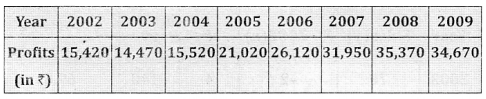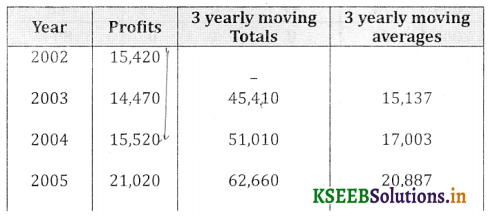Conclusion: The given time series data shows an upward trendQuestion 23.
For the following time series compute trend by five yearly moving averages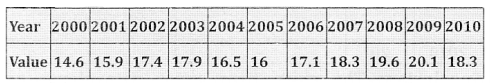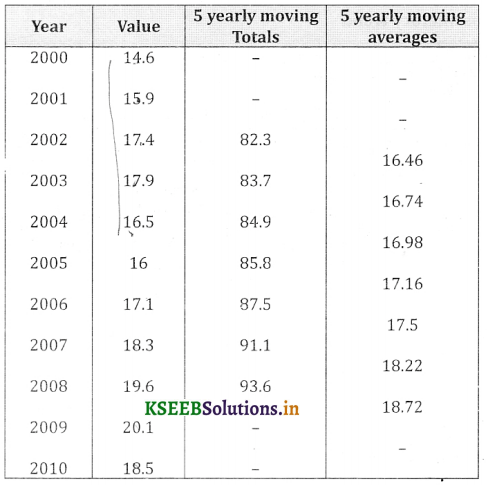Conclusion: The given time series data shows an upward trend

Question 24.
Calculate Trend values for the following data by using 5 yearly moving averages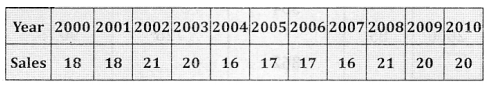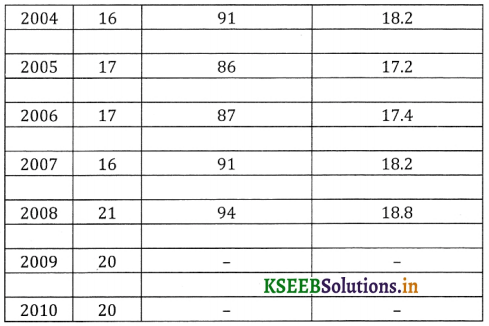Conclusion: The given time series data shows an oscillatory trendQuestion 25.
Calculate 4 yearly moving averages for the following dataConclusion: The given time Series data shows an a oscillatory trend.

Question 26.
Calculate 4 yearly moving averages for the following data.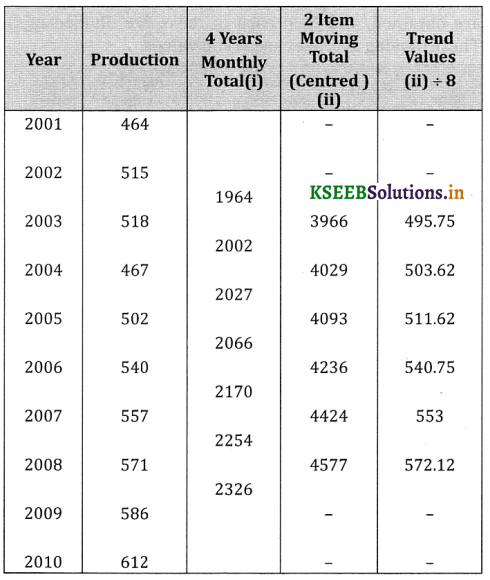Conclusion: The given time series data shows an upward trendQuestion 27.
Fit a straight line trend for the following data by the method of least squares and estimate the production of 2010.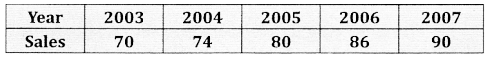Here n = 5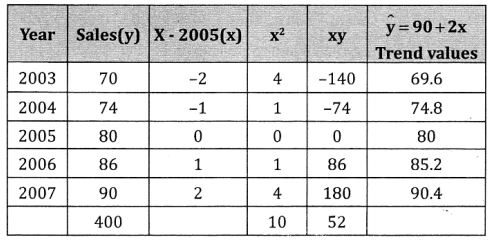(i) The straight line equation is given by y = a + bx
(ii) The normal equations are
Σy = na + b Σx
Σxy = a Σx + b Σx2
(iii) Since Σ x = 0(iv) Thus the trend equation is
Ŷ = a + bx
Ŷ = 80 + (5.2)x
(v) For the year 2010, x =10
∴ The estimated production for the year 2010 is
ŷ 2010 = 80 + 5.2 (10)
ŷ 2010 = 132.

Question 28.
Fit a straight line trend for the following data by the method of least squares and estimate the production for the year 2008Here n = 7(i) The straight line equation is given by y = a + b x
(ii) The normal equations are Σy = na + b Σ x
Σxy = aΣx + bΣx2
(iii) Since Σx= 0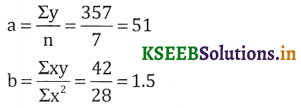(iv) Thus the trend equation is ŷ = a + bx
ŷ = 51 + 1.5x
(v) For the year 2008, x =5
∴ The estimated production for the year 2008 is
ŷ2008 = 51+ 1.5(5); ŷ2oo8= 58.5Question 29.
Below are given figures of production of a sugar factory. Apply the method of Least squares (Straight line) to determine the trend values(i) The straight line equation is given by y = a +bx
(ii) The normal equation are Σy = na + bΣx,
Σxy = aΣx + bΣx2
(iii)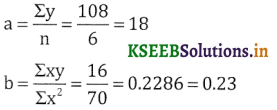(iv) The trend equation is ŷ = 18 + 0.23x
(v) By Substituting the values of x in trend equation we obtain the trend values

Question 30.
Fit a linear trend for the following time series and estimate the trend value 2010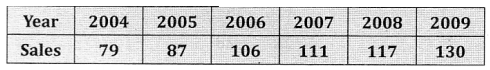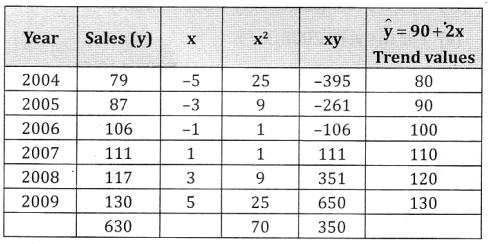(i) The straight line equation is given by y = a + bx
(ii) The normal equation are Σy = na + bΣx,
Σxy = aΣx + bΣx2
(iii) Since Σx = 0,(iv) The trend equation is ŷ = 105 + 5x
(v) For the year 2010, x = 7
∴ The estimated trend value is ŷ 2010 = 105 + 5(7)
ŷ 2010 = 140Question 31.
Find the trend values by least squares method (y = a + bx) from the following data and also estimate value for 2008Here n = 7(i) The equation of the straight line is y = a + bx
(ii) The normal equation are Σy = na + bΣx,
Σxy = aΣx + bΣx2
(iii) Since Σx = 0,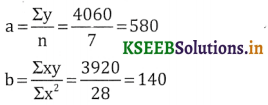(iv) The trend equation is ŷ = 580 + 140(x)
(v) For the year 2008, x = 5.
∴ The estimated value is ŷ2008= 580 + 140(5)
ŷ2oo8 = 1280

Question 32.
Below are given the figures of production of a sugar factory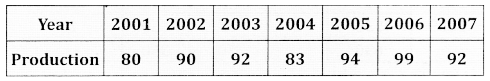(i) Fit a straight line trend by the method of least squares and obtain the trend values
(ii) Also estimate the production of the year 2008.
Here n = 7(i) The straight line equation is y = a + bx
(ii) The normal equation are Σy = na + bΣx,
Σxy = aΣx + bΣx2
(iii) Since Σx = 0,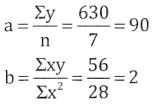(iv) The trend equation is ŷ = 90 + 2x
(v) By Substituting the values of x in trend equation we obtain the trend values
(vi) For the year 2008, x = 4.
∴ The estimated Production is ŷ2008 = 90 + 2x
= 90 + 2(4)
ŷ2008 = 98Question 33.
The productions of steel in million tons of a factory are given below. Apply the method of least squares to determine the trend values y = a + bx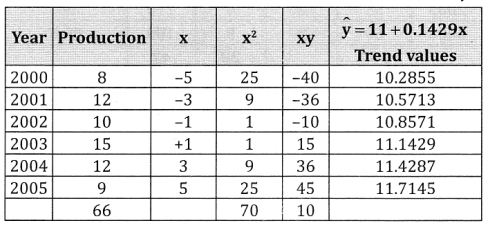Here n = 6
(i) The straight line equation is giver, by y = a + bx
(ii) The normal equation are Σy = na + bΣx,
Σxy = aΣx + bΣx2
(iii) since Σx = 0,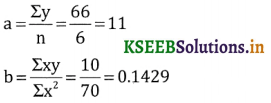(iv) The trend equation is ŷ = 11 + 0.1429x
(v) By Substituting the values of x in trend equation we obtain the trend values.

Question 34.
For the following time series data. Fit a curve of the type y = a + bx + cx2 estimate the value for the 2011.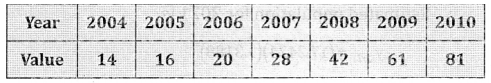The quadratic equation is y = a + bx + cx2
a, b and c are calculated by solving normal equations
Σy = na + bΣx + cΣx2 ….. (1)
Σxy = aΣx + bΣx2 + cΣx3 ….. (2)
Σx2y = aΣx2 + bΣx3 + cΣx4 ….. (3)
⇒ Substitute the values in 1, 2 and 3
7a + b(0) + c(28) = 262 ⇒ 7a + 28c = 262…(4) × 4
a(0) + b(28) + c(0) = 313 ⇒ 28b = 313 …(5)
28(a) + b(0) + c(196) = 1225 ⇒ 28a + 196c = 1225 …(6)
From equation (5)
b = $$\frac{313}{28}$$ = 11.1786 28
Solving equations (4) & (6), Multiply equation (4) by 4.
Now equation (4) – (6)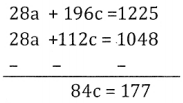c = $$\frac{177}{84}$$ = 2.1071
Substitute C value in equation (4)
7a + 28c = 262
7a + 28 (2.1071) = 262
7a + 58.9988 = 262
7a = 262 – 58.9988
7a = 203.0012
a = $$\frac{203.0012}{7}$$ = 29.0002
ŷ = a + bx + cx2 = 29.0002 + 11.1786x + 2.1071x2
For the year 2011, x = 4
∴ The estimated value for 2011 is
ŷ2011 = 29 + 11.1786(4) + 2.1071(16)
ŷ2011 = 107.42Question 35.
The following figures give the annual production of commodity. Estimate the out put in 2011. Use y = a + bx + cx2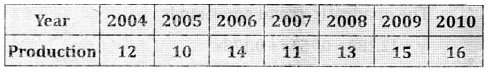(i) The quadratic equation is y = a + bx + cx2
The normal equations are
Σy = na + bΣx + cΣx2 …. (1)
Σxy = aΣx + bΣx2 + cΣx3 ….. (2)
Σx2y = aΣx2 + bΣx3 + cΣx4 …. (3)
Substitute the values in above equations
91 = 7a + b(0) + c(28) ⇒ 91 = 7a + 28c …. (4)
21 = a(0) + b(28) + c(0) ⇒ 21 = 28b
⇒ b = $$\frac{21}{28}$$ = 0.75
379 = a(28) + b(0) + c(196)
⇒ 379 = 28a + 196c ….. (5)
7a + 28c = 91 …. (4) × 4
28a + 196c = 379 …. (5)
Now equation (4) – (5)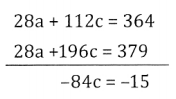c = $$\frac { 15 }{ 84 }$$ = 0.178
Substitute c value in equation (4)
7a + 28 (0.178) = 91
7a+ 4.984 =91
7a = 91 – 4.984
7a = 86.016
a = $$\frac { 86.016 }{ 7 }$$ = 12.228
ŷ = 12.288 + 0.751x + 0.178x2
For the year 2011, x = 4
∴ The estimated output in 2011 is
ŷ2011 =12.28 + 0.75(4) + 0.178(l6)
ŷ2011 = 18.14Question 36.
Population figures for a place are as given be low: Fit a curve of the type y = abx and estimate the population 2021.Here n = 5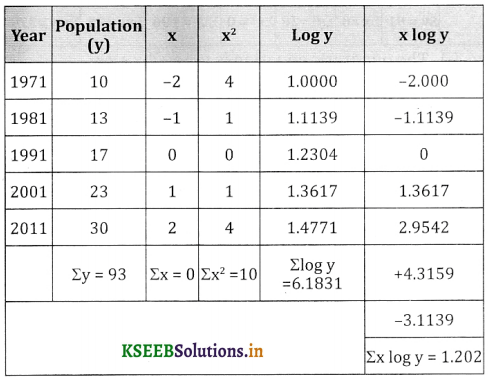(i) The equation of the exponential curve is y = abx
(ii) If Σx = 0, log a = $$\frac{\Sigma \log y}{n}=\frac{6.1831}{5}$$ = 1.2366
∴ a = antilog (1.2366) = 17.2424
and log b = $$\frac{\Sigma_{x} \log y}{\Sigma x^{2}}=\frac{1.202}{10}$$ = 0.1202
∴ b = antilog (0.1202) = 1.3188
∴ The trend equation is ŷ = (17.2424)(1.3188)x
For the year 2021, x = 3
∴ Estimated population for 2021 is
ŷ2021 =(17.2424)(1.3188)3
ŷ2021 = 39.55

Question 37.
The sales of a company for the years 2006 to 2012 are given below: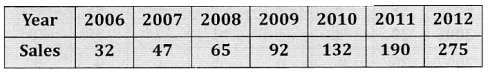Estimate sales figures for the year 2013. Using
y = abx, where x = year, y = sales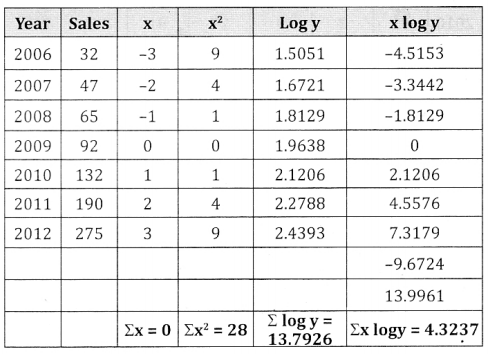(i) The equation of the exponential curve is y = abx
(ii) If Σx = 0,
loga = $$\frac{\Sigma \log y}{n}=\frac{13.7926}{7}$$ = 1.97
∴ a = antilog (1.97) = 93.32
and log b = $$\frac{\Sigma x \log y}{\Sigma x^{2}}=\frac{4.3237}{28}$$ = 0.1544
∴ b = antilog (0.1544) = 1.4269
∴ The trend equation is ŷ = (93.32)(1.42)x
For the year 2013, x = 4
∴ The estimated population for 2013 is
ŷ2o13 = (93.32)(1.42)4
ŷ2013 = 386.87### 2nd PUC Statistics Time Series Practical Assignments

Question 1.
For the following data find 3 yearly moving averagesThe given time series data shows are upward trend.

Question 2.
Calculate the trend values by finding three yearly moving averages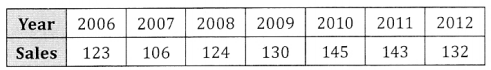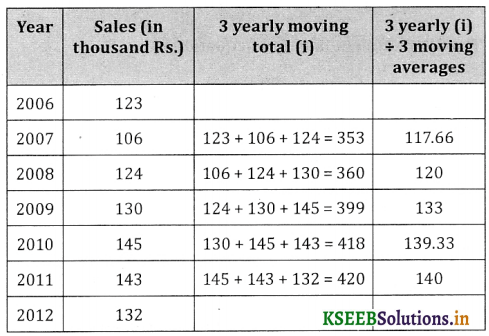The given time series data shows an upward trend.Question 3.
Compute 4 yearly moving averages for the following dataThe given time series data shows an oscillatory trend.

Question 4.
From the following data obtain the trend values by find four yearly moving averages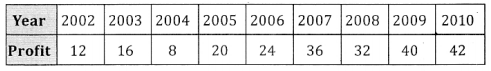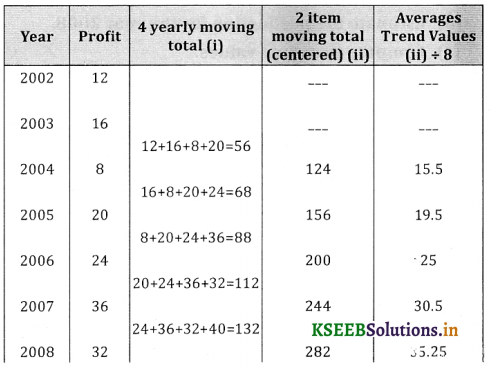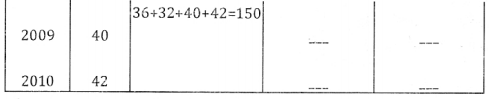The given time series data shows an upward trend.Question 5.
Compute five yearly moving averages for the following data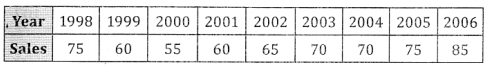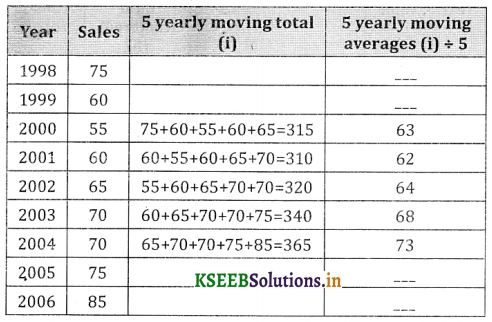The given time series data shows an upward trend.

Question 6.
Calculate the trend values by finding 5 yearly moving averages.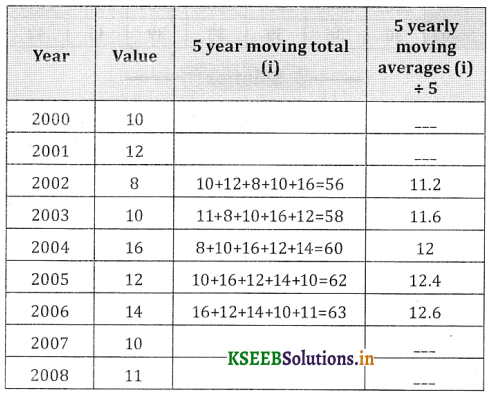The given time series data shows an upward trend.Question 7.
Below are given the figures of production (in thousand quintals) of a sugar factory.(a) Fit a straight line trend to these figures.
(b) Estimate the production for the year 2008.
(c) Compute the trend values.
Let X and Y be the year and production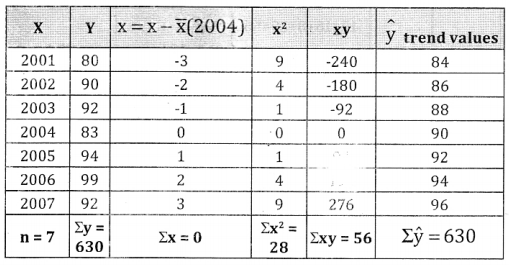From the normal equation : na + bΣx = Σy
since ΣX = 0; a = $$\frac{\Sigma y}{n}=\frac{630}{7}$$ = 90
From aΣx + bΣx2 = Σxy; b = $$\frac{\Sigma x y}{\Sigma x^{2}}=\frac{56}{28}$$ = 2
The fitted straight line trend equation is :
y = a + bx; ŷ = 90 + 2x
Estimation for 2008
x = 4, ŷ(2008) = 90 + 2(4) = 96(000’s) Tons.

Trend values:
For 2001; x = -3 ŷ (2001) = 90 + 2(-3) = 84 ,
ŷ(2002) = 90 + 2(-2) = 86
Similarly all other trend values an be calculated.

Question 8.
Production figures of a sugar factory in 1000 quintals are given below:(a) Fit a straight line trend to the above data.
(b) Plot these figures on a graph and show the trend line.
(c) Estimate the production for 2012.
Let X and Y be the year and production.c = 2 common difference between the years, x̄ = Middle most year.

From the normal equations :
na = bΣx = Σy since Σx = 0; a = $$\frac{\Sigma y}{n}=\frac{91}{7}$$ =13
From aΣx = bΣx2 = bΣx2 = Σxy ; b = $$\frac{\Sigma x y}{\Sigma x^{2}}=\frac{21}{28}$$ = 0.75

The fitted straight line trend equation is :
y = a + bx; ŷ = 13 + 0.75 x
Estimation for 2008:
x = 4, ŷ(2012) = 13 +0.75(4) = 13 + 6 = 16
Trend vaiues : For 1998 ;
x = -3; ŷ (l998) = 13 + 0.75(-3)
= 13 – 2.25 = 10.75 units.
Similarly for 2000, ŷ(2000) = 13 + 0.75(-2) = 11.5 and others can be calculated.

GRAPH OF PRODUCTION TIME SERIESFrom the trend line, the time series / production shows upward / increasing trend.Question 9.
Following data gives the population of city.
a. Fit a linear trend and find the trend values.
b. Estimate the population in 2011.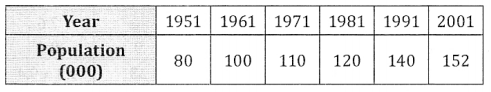Let x and y be the year and population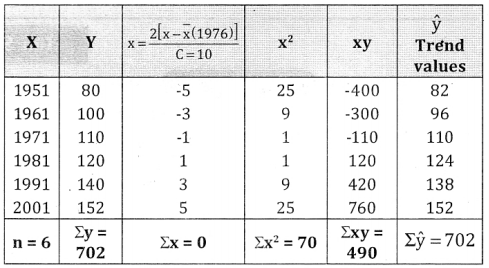C = 10, common difference between the years.
x̄ = $$\frac{1971+1981}{2}$$ = 1976
From the normal equation :
na + bΣx = Σy since Σx = 0; a = $$\frac{\Sigma y}{n}=\frac{702}{6}$$ = 1.17
From aΣx + bΣx2 = Σxy ; b = $$\frac{\Sigma x y}{\Sigma x^{2}}=\frac{490}{70}$$ = 7

(a) The fitted straight linear trend equation is :
y = a + bx ; ŷ = 117 + 7x
Trend values : For 1951;
x = -5; ŷ = 117 + 71.55 = 117-35 = 82
Similarly compute for other years. Ie.
ŷ(1961) = 117 + 7(-3) = 117 – 21 = 96 etc.

(b) Estimation for 2011; x = 7;
ŷ(2011) = 117 + 7(7)
= 117 + 49 = 166(000’s)

Question 10.
Fit y = a + bx form the straight line trend to the data, plot the original and trend values on graph.From the normal equation : na + bΣx = Σy
since Σx = 0; a$$\frac{\Sigma y}{n}=\frac{48}{6}$$ = 8
From : aΣx + bΣx2 = Σxy ; b = $$\frac{\Sigma x y}{\Sigma x^{2}}$$ = $$\frac{30}{70} 0.4286$$
The fitted straight line trend equation is :
y = a + bx ,i.e, ŷ = 8 + 0.4286x
Trend values are : q0 = $$\frac{v_{0}}{p_{0}}$$
ŷ(2003) = 8 + 0.4286(-3) = 7.6
Similarly all other trend values is can be computed.

Graph of Time Seriesfrom the trend line the time series show upward trendQuestion 11.
Fit y = a + bx form the straight line trend to the data, plot the original and trend values on graph.Let X and Y be the year and profit.
The parabolic equation is : y = a+ bx + cx2
The normal equations are :
na + bΣx + cΣx2 = Σy ….. (1)
aΣx + bΣx2 + cΣx2 = Σxy ….. (2)
aΣx2 + bΣx3 + cΣx4 = Σx2y ….. (3)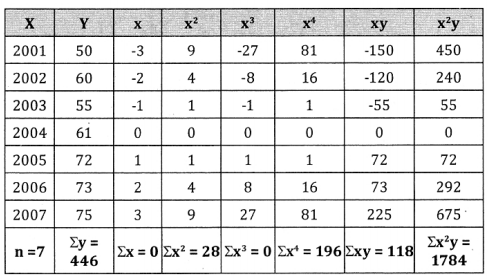From equation (1): 7a + b.0 + c.28 = 446
7a + 28c = 446 ….. (4)
From equation (2) : a.0 + b.28 + c.0 =118
28b = 118 ; b = $$\frac{118}{28}$$ = 4.214
From equation (3) : a.28 + n.0 + c.196 = 1784
28a + 196c = 1784 …(5)
To get ‘a’ and ‘c’ solve (4) and (5)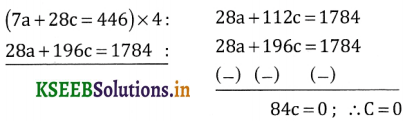Put C = 0 in (4) 7a + 28 (0) = 446 ; 7a=446;
a = $$\frac{446}{7}$$ = 63.714
The fitted parabolic trend equation is
ŷ = 63.714 + 4.214 x + 0x2
ŷ = 63.714 + 4.214x
Estimation for the year 2010; x = 6;
ŷ(2010) = 63.714 + 4.214(6) = 88.998

Question 12.
For the following time series fit a parabolic trend of the type y = a + bx + cx2 by the method of least squares. Estimate the production in 2009.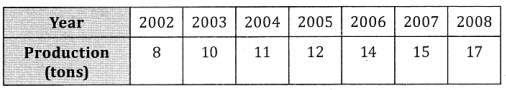Let X and Y be the year and productionFrom the normal equations : na + bΣx + cΣx2 = Σy
7a + b.0 + c.28 = 87
7a + 28c = 87 …(1)
From : aΣx + bΣx2 + cΣx3 = Σxy
a.0 + b.28 + c.0 = 40 ; b = $$\frac{40}{28}$$ = 1.43 28
And from : aΣx2 + bΣx3 + cΣx4 = Σx2y
a.28 + b.O + c.196 = 350
28a + 196c = 350 …… (2)∴ c = $$\frac{2}{84}$$ = o 024 Put c = 0-024 in equation (1)
We get 7a + 28 (0.024) = 87 ; 7a + 0.672 = 87
7a = 87 – 0.672; ∴ a = $$\frac{86.328}{7}$$ = 12.333
The fitted parabolic trend equation is : y = a + bx + cx2
ŷ = 12.333 + 1.43x + 0.024x2
Estimation for the year 2009 ; x = 4, x2 = 42 = 16
ŷ(2009) = 12.333 + 1.43(4) + 0.024(16) = 18.437 tons.Question 13.
Fit a second degree equation of the form y = a + bx + cx2 to the following data regarding profits and estimate the profit for the year 2010.Let X and Y be the year and productionFrom the normal equations : na + bΣx + cΣx2 = Σy
4a + b.0 + c.20 = 45
4a + 20c = 45 …(1)
From: aΣx + bΣx2 + cΣx3 = Σxy
a.20 + b.20 + c.0 = 1
20b = 1; b = $$\frac { 1 }{ 20 }$$ = 0.05
And from : aΣx2 + bΣx3 + cΣx4 = Σx2y
a.20 + b.0 + c.164 = 205
20a + 164c = 205 …(2)∴ c = $$\frac { 20 }{ -64 }$$
Put c = – 0.3125 in equation (1)
We get 4a + 20 (-0.3125) = 45; 4a – 6.25 = 45
4a = 45 + 6.25; ∴ a = $$\frac { 51.25 }{ 4 }$$ = 12.8125
The fitted parabolic trend equation is : y = a + bx + cx2
ŷ = 12.8125 + 0.05x + 0.3125x2
Estimation for the year 2010 ; x = 5 ; x2 = 25
ŷ(2010) = 12.8125 + 0.05(5) – 0.3125(25)
= 5.25(000’s)Question 14.
The following table gives the profits of a concern for 5 years ending 2009.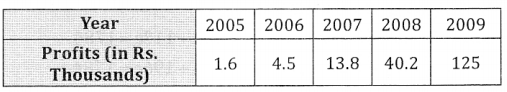Fit an equation of the type y = abx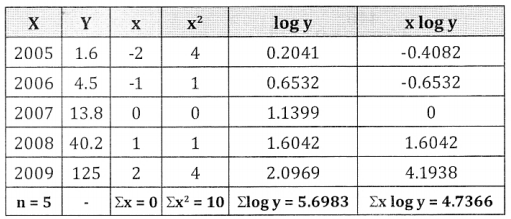log a = $$\frac{\Sigma \log \mathrm{y}}{\mathrm{n}}=\frac{5.6983}{5}$$ = 1.1397
log = $$\frac{\Sigma_{x} \log y}{\Sigma x^{2}}$$ = $$\frac{4.7366}{10}$$ =0.4737